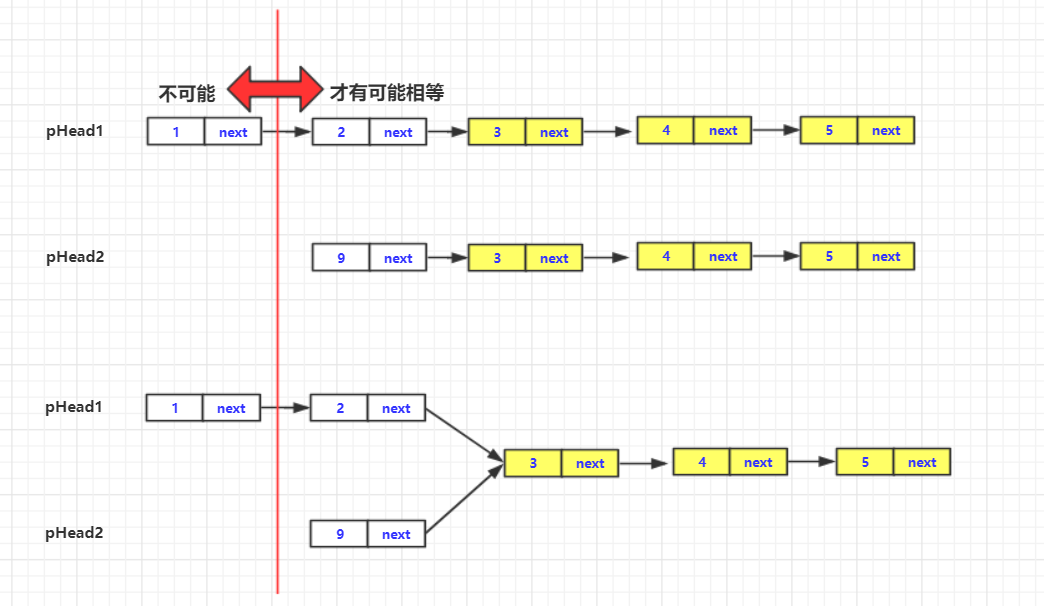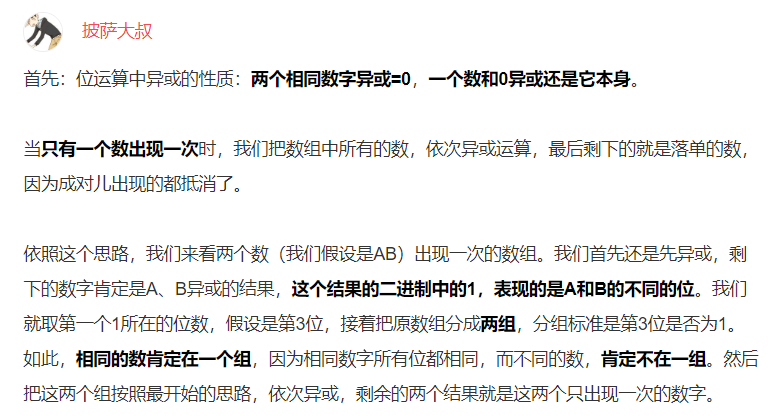# 剑指offer(34-40题)详解

### 文章目录

欢迎关注个人数据结构专栏
剑指offer系列
剑指offer(1-10题)详解
剑指offer(11-25题)详解
剑指offer(26-33题)详解
剑指offer(34-40题)详解
剑指offer(51-59题)详解
剑指offer(34-40题)详解
微信公众号：bigsai声明：大部分题基本未参考题解，基本为个人想法，如果由效率太低的或者错误还请指正！，如果有误导，还请指正！

## 34 第一个只出现一次的字符

题目描述

在一个字符串(0<=字符串长度<=10000，全部由字母组成)中找到第一个只出现一次的字符,并返回它的位置, 如果没有则返回 -1（需要区分大小写）

思路
用hashmap储存记录每个字母出现的次数。然后再进行遍历查找第一个出现一次的字母返回位置。

实现代码：

import java.util.HashMap;
public class Solution {
public  int FirstNotRepeatingChar(String str) {
HashMap<String, Integer>map=new HashMap<String, Integer>();
String team="";
for(int i=0;i<str.length();i++)
{
team=str.charAt(i)+"";
if(map.containsKey(team))
{
map.put(team, 2);
}
else {
map.put(team, 1);
}
}
int index=-1;
for(int i=0;i<str.length();i++)
{
team=str.charAt(i)+"";
if(map.get(team)==1)
{
index=i;
break;
}
}
return index;
}
}


## 35 数组中的逆序数

题目描述

在数组中的两个数字，如果前面一个数字大于后面的数字，则这两个数字组成一个逆序对。输入一个数组,求出这个数组中的逆序对的总数P。并将P对1000000007取模的结果输出。 即输出P%1000000007
输入描述:
题目保证输入的数组中没有的相同的数字

数据范围

对于%50的数据,size<=10^4

对于%75的数据,size<=10^5

对于%100的数据,size<=2*10^5


示例1

输入
1,2,3,4,5,6,7,0
输出
7

思路
至于逆序数，一般有三种方法求解，首当其中的就是暴力的O(n2)的方法，但是这种方法复杂度过高一看这个数据范围肯定是过不了的。18年夏天遇到过逆序数记录下来，但是写的不够好，从写了一下！

今天刚写归并排序和逆序数各位一定好好看看，进行了详细分析。

然后可以采用树状数组或者归并排序求解逆序数。当然，笔者这里采用的是归并排序。

实现代码为：

public class Solution {
static int value=0;
public static int InversePairs(int [] array) {
mergesort(array,0,array.length-1);
return value;

}
private static void mergesort(int[] array, int l, int r) {
int mid=(l+r)/2;
if(l<r)
{
mergesort(array, l, mid);
mergesort(array, mid+1, r);
merge(array, l,mid, r);
}
}

private static void merge(int[] array, int l, int mid, int r) {

int lindex=l;int rindex=mid+1;
int team[]=new int[r-l+1];
int teamindex=0;
while (lindex<=mid&&rindex<=r) {
if(array[lindex]<=array[rindex])
{
team[teamindex++]=array[lindex++];
}
else {
team[teamindex++]=array[rindex++];
value+=mid-lindex+1;
value%=1000000007;
}
}
while(lindex<=mid)
{
team[teamindex++]=array[lindex++];

}
while(rindex<=r)
{
team[teamindex++]=array[rindex++];
}
for(int i=0;i<teamindex;i++)
{
array[l+i]=team[i];
}
}
}


## 36 两个链表的第一个公共节点

题目描述

输入两个链表，找出它们的第一个公共结点

思路
这题的吧不要用暴力匹配的O(n2)的方法。大家直到链表是一条直的对吧？然后一个链表节点相同也就是后面都相同是吧？ 所以如果有相同节点的话那么一定从那个节点到最后都是相同的。并且肯定在短的后面才可能匹配。

至于实现方式其实还是蛮多的，比如你可以先遍历一次分别把两个长度求出来然后把长的节点往后移到相同长度，然后一个个比较就好。当然这样可能跑2次但是影响确实不大。你也可以借助外部空间然后将遍历一次的存下来。然后从后往前找到第一个不一样的那个后面就是了。 当然笔者采用上面一个方法并没有采用外部空间。实现代码为：

/*
public class ListNode {
int val;
ListNode next = null;

ListNode(int val) {
this.val = val;
}
}*/
public class Solution {
while (lp1>lp2) {
}
while (lp1<lp2) {
}
}
}
int len=0;
while (team!=null) {
team=team.next;
len++;
}
return len;
}
}


## 37 数字在排序数组中出现的次数

题目描述

统计一个数字在排序数组中出现的次数

思路
数组问题，一般枚举能过但是不够优雅。有序的数组、序列当然可以使用二分法哈！先二分找到其中任意一个数字所在的序号，然后根据这个坐标左右试探就可以直到多少个啦！

实现代码为：

public class Solution {
public int GetNumberOfK(int [] array , int k) {
int l=0,r=array.length;
int mid=(l+r)/2;
while (l<r) {
if(array[mid]==k)
{
return search(array,mid);
}
else if (array[mid]<k) {
l=mid+1;mid=(l+r)/2;
}
else {
r=mid;mid=(l+r)/2;
}
}
return 0;
}

private int search(int[] array, int mid) {
int l=mid-1,r=mid+1;
int value=0;
while (l>=0&&array[l]==array[mid]) {
value++;l--;
}
while (r<array.length&&array[r]==array[mid]) {
value++;r++;
}
return value+1;
}
}


## 38 二叉树的深度

题目描述

输入一棵二叉树，求该树的深度。从根结点到叶结点依次经过的结点（含根、叶结点）形成树的一条路径，最长路径的长度为树的深度

思路
因为二叉树有 左右子节点，左右节点的问题。每个孩子都要比自己更深一层，对于这个问题，你可以自己定义一个包含次数的结构体或者类用队列或者栈进行遍历找到最大的。但是这题可以直接使用递归进行求解。父节点和子节点之间的关系就是deep(parent)=max(parent.left,parent.right)+1。当然，这里的left和right节点也要判空之类的处理。

实现代码为：

/**
public class TreeNode {
int val = 0;
TreeNode left = null;
TreeNode right = null;

public TreeNode(int val) {
this.val = val;
}
}
*/
public class Solution {
public int TreeDepth(TreeNode root) {
if(root==null)return 0;
return Math.max(TreeDepth(root.left), TreeDepth(root.right))+1;
}
}


## 39 平衡二叉树

题目描述

输入一棵二叉树，判断该二叉树是否是平衡二叉树。

思路
至于平衡二叉树(avl)，之所以平衡，是因为它的任意一个左右节点的高度相差都小于等于1. 如果有不了解的可以参考以前写的一篇avl二叉平衡树 。但是这个跟文中写的的其实不太一样，是因为之前我创造二叉树的时候有个height值每次插入的时候动态维护，判断是否平衡。但是显然这题什么都没有，需要自己完成所有

好吧，来就来，不就是判断每个节点都要满足左右高度都要相差在1之内嘛！上面那题，不就是求高度的嘛？！！我用个队列把所有点存下来，然后一个个判断可还行？虽然可能效率不一定高。但是还是能过的。

实现代码为：

import java.util.ArrayDeque;
import java.util.Queue;
public class Solution {
public boolean IsBalanced_Solution(TreeNode root) {
if(root==null)return true;
Queue<TreeNode>q1=new ArrayDeque<TreeNode>();
while (!q1.isEmpty()) {
TreeNode team=q1.poll();
if(Math.abs(TreeDepth(team.left)-TreeDepth(team.right))<=1)
{
}
else {
return false;
}
}
return true;
}

public int TreeDepth(TreeNode root) {
if(root==null)return 0;
return Math.max(TreeDepth(root.left), TreeDepth(root.right))+1;
}
}


## 40 数组中只出现一次的数字

题目描述

一个整型数组里除了两个数字之外，其他的数字都出现了两次。请写程序找出这两个只出现一次的数字。

思路
这题只能用普通方法过，但是还是有技巧的。感觉可以用位运算但是奈何自己没想出来。两个相同的数进行异或^运算等于0.而0和任何数异或等于它自己。如果只求1个数那可能好搞一些，但是后面会研究讨论区大佬的位运算方案

对于普通思路，可能大部分人会用hashmap先添加，最后遍历两个次数等于1个的两个数返回。但是其实可以使用hash进行减。如果存在这个元素，就减去这个元素。如果不存在那就加入hash中，那么最终剩的2个元素其实就是我们出现一次的元素。由于java底层用hashmap维护hashset我就用hashmap了差不了太大。

实现代码为：


import java.util.HashMap;
//num1,num2分别为长度为1的数组。传出参数
//将num1,num2设置为返回结果
public class Solution {
public void FindNumsAppearOnce(int [] array,int num1[] , int num2[]) {
HashMap<Integer, Integer>map=new HashMap<Integer, Integer>();
for(int i=0;i<array.length;i++)
{
if(map.containsKey(array[i])) {map.remove(array[i]);}
else {
map.put(array[i],1);
}
}
int count=0;
for(Integer va:map.keySet())
{
if(count++==0)num1=va;
else {
num2=va;
}
}
}
}


参考讨论区：
这题的讨论区很妙，用^来解决问题。至于讨论区的题解稍微解释一下：大概就是将数组一分为2份
就是说先异或到最后得到一个数，肯定是a^b的值。这个数位位1的那个肯定就是两个位不同的。比如10100.第三位就是a和b不同的，然后我们就再次遍历所有数，用两个数进行异或，如果第三位为1xx那么和a1异或，如果为0xx那么和a2异或。就相当于把这个数逻辑一分为2.因为所有数除了这两都出现两次，所以是可以消下去的！实现代码为：

链接：https://www.nowcoder.com/questionTerminal/e02fdb54d7524710a7d664d082bb7811?f=discussion
来源：牛客网

public class Solution {
public void FindNumsAppearOnce(int[] array, int[] num1, int[] num2)    {
int length = array.length;
if(length == 2){
num1 = array;
num2 = array;
return;
}
int bitResult = 0;
for(int i = 0; i < length; ++i){
bitResult ^= array[i];
}
int index = findFirst1(bitResult);
for(int i = 0; i < length; ++i){
if(isBit1(array[i], index)){
num1 ^= array[i];
}else{
num2 ^= array[i];
}
}
}

private int findFirst1(int bitResult){
int index = 0;
while(((bitResult & 1) == 0) && index < 32){
bitResult >>= 1;
index++;
}
return index;
}

private boolean isBit1(int target, int index){
return ((target >> index) & 1) == 1;
}
}


欢迎关注公众号：bigsai 长期奋战输出！

还能输入1000个字符
©️2019 CSDN 皮肤主题: 代码科技 设计师: Amelia_0503扫一扫，手机浏览